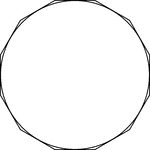### Dodecagon Circumscribed About A Circle

Illustration of a dodecagon circumscribed about a circle. This can also be described as a circle inscribed…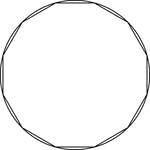### Dodecagon Inscribed In A Circle

Illustration of a dodecagon inscribed in a circle. This can also be described as a circle circumscribed…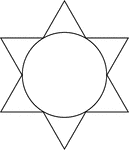### Star Circumscribed About A Circle

Illustration of a 6-point star (convex dodecagon) circumscribed about a circle. This can also be described…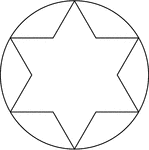### Star Inscribed In A Circle

Illustration of a 6-point star (convex dodecagon) inscribed in a circle. This can also be described…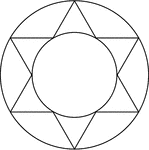### Star Inscribed And Circumscribed About Circles

Illustration of a 6-point star (convex dodecagon) inscribed in a large circle and circumscribed about…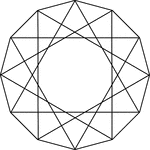### 12 Triangles Inscribed In A Dodecagon

Illustration of 12 equilateral triangles inscribed in an equilateral dodecagon. Each vertex of the dodecagon…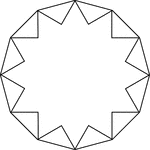### 12-Point Star Inscribed In A Dodecagon

Illustration of a 12-point star (24-sided polygon) inscribed in a regular dodecagon. This can also be…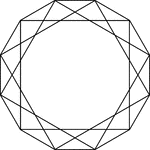### 3 Square Inscribed In A Dodecagon

Illustration of 3 squares inscribed in an regular dodecagon. Each vertex of the dodecagon is also a…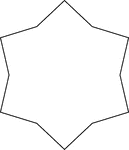### Concave Equilateral Dodecagon

A 6-point star made from a non-regular concave dodecagon in which all sides are equal in length. There…### Hexagon And Triangle Inscribed In A Dodecagon

Illustration of a regular hexagon and an equilateral triangle inscribed in a regular dodecagon. This…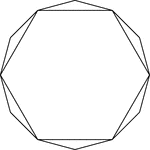### Hexagon Inscribed In A Dodecagon

Illustration of a regular hexagon inscribed in a regular dodecagon. This could also be described as…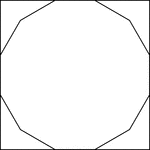### Square Circumscribed About A Dodecagon

Illustration of a square circumscribed about a regular dodecagon. This could also be described as a…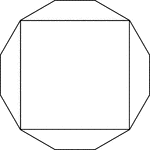### Square Inscribed In A Dodecagon

Illustration of a square inscribed in an regular dodecagon. This could also be described as a regular…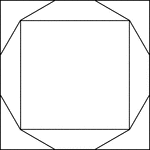### Squares Inscribed and Circumscribed About a Regular Dodecagon

Illustration of 2 squares; one inscribed in a regular dodecagon and the other circumscribed about the…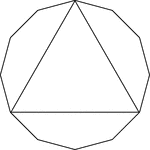### Triangle Inscribed In A Dodecagon

Illustration of an equilateral triangle inscribed in an equilateral dodecagon. This could also be described…